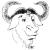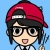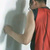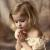## C++11 中值得关注的几大变化（详解）

#### Lambda 表达式

Lambda表达式来源于函数式编程，说白就了就是在使用的地方定义函数，有的语言叫“闭包”。表达式的简单语法如下，我在这里就不再讲这个事了。

`[capture](parameters)->return_type {body}`

```int main()
{
char s[]="Hello World!";
int Uppercase = 0; //modified by the lambda
for_each(s, s+sizeof(s), [&Uppercase] (char c) {
if (isupper(c))
Uppercase++;
});
cout << Uppercase << " uppercase letters in: " << s <<endl;
}```

```template <class T>
class less
{
public:
bool operator()(const T&l, const T&r)const
{
return l < r;
}
};```

#### 自动类型推导 auto

```auto x=0; //x has type int because 0 is int
auto c='a'; //char
auto d=0.5; //double
auto national_debt=14400000000000LL;//long long```

auto 最大的好处就是让代码简洁，尤其是那些模板类的声明，比如：STL中的容器的迭代子类型。

`vector<int>::const_iterator ci = vi.begin();`

`auto ci = vi.begin();`

`auto obj = ProcessData(someVariables);`

`auto pObject = new SomeType<OtherType>::SomeOtherType();`

#### 自动化推导 decltype

1. 如果表达式e是一个变量，那么就是这个变量的类型。
2. 如果表达式e是一个函数，那么就是这个函数返回值的类型。
3. 如果不符合1和2，如果e是左值，类型为T，那么decltype(e)是T&；如果是右值，则是T。

```const vector<int> vi;
typedef decltype (vi.begin()) CIT;
CIT another_const_iterator;```

```decltype(&myfunc) pfunc = 0;

typedef decltype(&A::func1) type;```

#### auto 和 decltype 的差别和关系

Wikipedia 上是这么说的（关于decltype的规则见上）

```#include <vector>

int main()
{
const std::vector<int> v(1);
auto a = v;        // a 的类型是 int
decltype(v) b = 1; // b 的类型是 const int&, 因为函数的返回类型是
// std::vector<int>::operator[](size_type) const
auto c = 0;           // c 的类型是 int
auto d = c;           // d 的类型是 int
decltype(c) e;        // e 的类型是 int, 因为 c 的类型是int
decltype((c)) f = c;  // f 的类型是 int&, 因为 (c) 是左值
decltype(0) g;        // g 的类型是 int, 因为 0 是右值
}```

```template< typename LHS, typename RHS>
auto AddingFunc(const LHS &lhs, const RHS &rhs) -> decltype(lhs+rhs)
{return lhs + rhs;}```

#### 统一的初始化语法

C/C++的初始化的方法比较，C++ 11 用大括号统一了这些初始化的方法。

```int arr={0,1,2,3};
struct tm today={0};```

```struct A { int m; }; // POD
struct B { ~B(); int m; }; // non-POD, compiler generated default ctor
struct C { C() : m() {}; ~C(); int m; }; // non-POD, default-initialising m```

POD的初始化有点怪，比如上例，new A; 和new A(); 是不一样的，对于其内部的m，前者没有被初始化，后者被初始化了（不同 的编译器行为不一样，VC++和GCC不一样）。而非POD的初始化，则都会被初始化。

```C c {0,0}; //C++11 only. 相当于: C c(0,0);

int* a = new int { 1, 2, 0 }; /C++11 only

class X {
int a;
public:
X() : a{1,2,3,4} {} //C++11, member array initializer
};```

```// C++11 container initializer
vector<string> vs={ "first", "second", "third"};
map singers =
{ {"Lady Gaga", "+1 (212) 555-7890"},
{"Beyonce Knowles", "+1 (212) 555-0987"}};```

```class C
{
int a=7; //C++11 only
public:
C();
};```

#### Deleted 和 Defaulted 函数

```struct A
{
A()=default; //C++11
virtual ~A()=default; //C++11
};```

```struct NoCopy
{
NoCopy & operator =( const NoCopy & ) = delete;
NoCopy ( const NoCopy & ) = delete;
};
NoCopy a;
NoCopy b(a); //compilation error, copy ctor is deleted```

```struct SomeType
{
SomeType() = default; // 使用编译器生成的默认构造函数
SomeType(OtherType value);
};```

1）让你的对象只能生成在栈内存上：

```struct NonNewable {
void *operator new(std::size_t) = delete;
};```

2）阻止函数形参的类型：（若尝试以 double 的形参调用 `f()`，将会引发编译期错误， 编译器不会自动将 double 形参转型为 int 再调用`f()`

```void f(int i);
void f(double) = delete;```

#### nullptr

C/C++的NULL宏是个被有很多潜在BUG的宏。因为有的库把其定义成整数0，有的定义成 (void*)0。在C的时代还好。但是在C++的时代，这就会引发很多问题。你可以上网看看。这是为什么需要 `nullptr` 的原因。 `nullptr` 是强类型的。

```void f(int); //#1
void f(char *);//#2
//C++03
f(0); //二义性
//C++11
f(nullptr) //无二义性，调用f(char*)```

`所以在新版中请以 nullptr` 初始化指针。

#### 委托构造

```class SomeType {
private:
int number;
string name;
SomeType( int i, string&amp; s ) : number(i), name(s){}
public:
SomeType( )               : SomeType( 0, "invalid" ){}
SomeType( int i )         : SomeType( i, "guest" ){}
SomeType( string&amp; s ) : SomeType( 1, s ){ PostInit(); }
};```

```class BaseClass
{
public:
BaseClass(int iValue);
};

class DerivedClass : public BaseClass
{
public:
using BaseClass::BaseClass;
};```

#### 右值引用和move语义

```void naiveswap(string &amp;a, string &amp;b)
{
string temp = a;
a=b;
b=temp;
}```

C++ 11增加一个新的引用（reference）类型称作右值引用（R-value reference），标记为typename &&。他们能够以non-const值的方式传入，允许对象去改动他们。这项修正允许特定对象创造出move语义。

```class string
{
string (string&&); //move constructor
string&& operator=(string&&); //move assignment operator
};```

The C++11 STL中广泛地使用了右值引用和move语议。因此，很多算法和容器的性能都被优化了。

#### C++11 STL 标准库

C++ STL库在2003年经历了很大的整容手术 Library Technical Report 1 (TR1)。 TR1 中出现了很多新的容器类 (`unordered_set`, `unordered_map`, `unordered_multiset`, 和 `unordered_multimap`) 以及一些新的库支持诸如：正则表达式， tuples，函数对象包装，等等。 C++11 批准了 TR1 成为正式的C++标准，还有一些TR1 后新加的一些库，从而成为了新的C++ 11 STL标准库。这个库主要包含下面的功能：

##### 新型智能指针

C++98 的知能指针是 `auto_ptr， 在C++ 11中被废弃了。`C++11  引入了两个指针类： shared_ptrunique_ptr。 shared_ptr只是单纯的引用计数指针，`unique_ptr 是用来取代auto_ptr`。 `unique_ptr` 提供 `auto_ptr` 大部份特性，唯一的例外是 `auto_ptr` 的不安全、隐性的左值搬移。不像 `auto_ptr``unique_ptr` 可以存放在 C++0x 提出的那些能察觉搬移动作的容器之中。

##### 新的算法

```#include <algorithm>
//C++11 code
//are all of the elements positive?
all_of(first, first+n, ispositive()); //false
//is there at least one positive element?
any_of(first, first+n, ispositive());//true
// are none of the elements positive?
none_of(first, first+n, ispositive()); //false```

```#include <algorithm>
int source={0,12,34,50,80};
int target;
//copy 5 elements from source to target
copy_n(source,5,target);```

```include <numeric>
int a={0};
char c={0};
iota(a, a+5, 10); //changes a to {10,11,12,13,14}
iota(c, c+3, 'a'); //{'a','b','c'}```

（全文完）

00c++ 无疑是世界上最复杂的编程语言, 这样会吓退很多初学者啊...
0C++不愧是世界上最复杂的编程语言，没有之一
0C＋＋真是从海洋深处到火星表面，通杀。

0